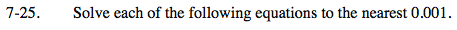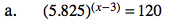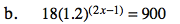Home > INT3 > Chapter 7 > Lesson 7.1.2 > Problem7-25

7-25.
1. Solve each of the following equations to the nearest 0.001. Homework Help ✎

1. (5.825)(x–3) = 120

2. 18(1.2)(2x–1) = 900Since you are solving for an exponent, take the log of each side:

Apply the Power Property of Logarithms:

Divide both sides of the equation by log(5.825):

Add 3 to both sides and use your calculator.
Remember to round to the nearest 0.001.

log(5.825)(x − 3) = log(120)

(x − 3)log(5.825) = log(120)

$\frac{(x-3) \text{log}(5.825)}{ \text{log}(5.825)} = \frac{ \log (120)}{ \log (5.825)}$Divide both sides of the equation by 18.
Then follow the steps in part (a).